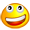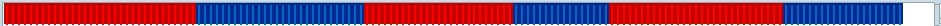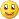# W D Gann Indicators and others - page 26154266

ismael360:
Hi Mladen does the oscillator have super smoother included with in it? ish

ish

It does not. The oscillator is made as a simple difference of price and Gann T3 high low activator value748

ish It does not. The oscillator is made as a simple difference of price and Gann T3 high low activator value

This is probably a strange question but, is it possible to add super smoothing to the Difference of Price in the Gann T3 High Low Oscillator??

ish154266

ismael360:
This is probably a strange question but, is it possible to add super smoothing to the Difference of Price in the Gann T3 High Low Oscillator?? ish

No problem

Here is a version that uses super smoother for result smoothing. To turn it off, set the Smooth to <= 1748

No problem Here is a version that uses super smoother for result smoothing. To turn it off, set the Smooth to <= 1

ish113

Thank you so much Mladen..I appreciated for this...Super smoother one is fine.Thank you again..Take care and have plenty of pips!352

Hi Guys,

Does someone have the Gann Trend Oscillator ?

Thanks a lot and in advance

Have a nice day

Zilliq154266

zilliq:
Hi Guys,

Does someone have the Gann Trend Oscillator ?

Thanks a lot and in advance

Have a nice day

Zilliq

Haven't seen one so far352

Yes I haven't find it, so I code it but on PRT. If my code can help someone to translate it on MT4Did you see my request about "RMX" pleazzzz ?

Thanks a lot and have a nice day

Zilliqa1= average[p](high)

b1=average[p](low)

if (close crosses over a1) and tend1 then

mini=lowest(low)

tend=1

elsif (close crosses under b1) and tend-1 then

maxi=highest(high)

tend=-1

else

if barindex>1 then

mini=mini

maxi=maxi

tend=tend

else

tend=1

endif

endif

if close crosses over maxi then

trenda=1

trendb=0

elsif close crosses under mini then

trendb=1

trenda=0

else

trenda=trenda

trendb=trendb

endif

return maxi as "maxi", mini as "mini", trenda as "Tendance haussière", trendb as "Tendance baissière"

Files:154266

zilliq:

Yes I haven't find it, so I code it but on PRT. If my code can help someone to translate it on MT4Did you see my request about "RMX" pleazzzz ?

Thanks a lot and have a nice day

Zilliqa1= average[p](high)

b1=average[p](low)

if (close crosses over a1) and tend1 then

mini=lowest(low)

tend=1

elsif (close crosses under b1) and tend-1 then

maxi=highest(high)

tend=-1

else

if barindex>1 then

mini=mini

maxi=maxi

tend=tend

else

tend=1

endif

endif

if close crosses over maxi then

trenda=1

trendb=0

elsif close crosses under mini then

trendb=1

trenda=0

else

trenda=trenda

trendb=trendb

endif

return maxi as "maxi", mini as "mini", trenda as "Tendance haussière", trendb as "Tendance baissière"

zilliq

Where is that formula from?

I am asking it since it looks very similar to Gann high low activator (as far as I can understand the prt code)352

From me MladenYou're right, in fact we need first to define the Hilo activator, who will define the peak and valley when there is a reversal as Krausz/Gann define it

When the close cross over the last peak (maxi), the trend is up, and when the indicator cross under the last valley (mini) the trend is down

Have a nice day

Zilliq